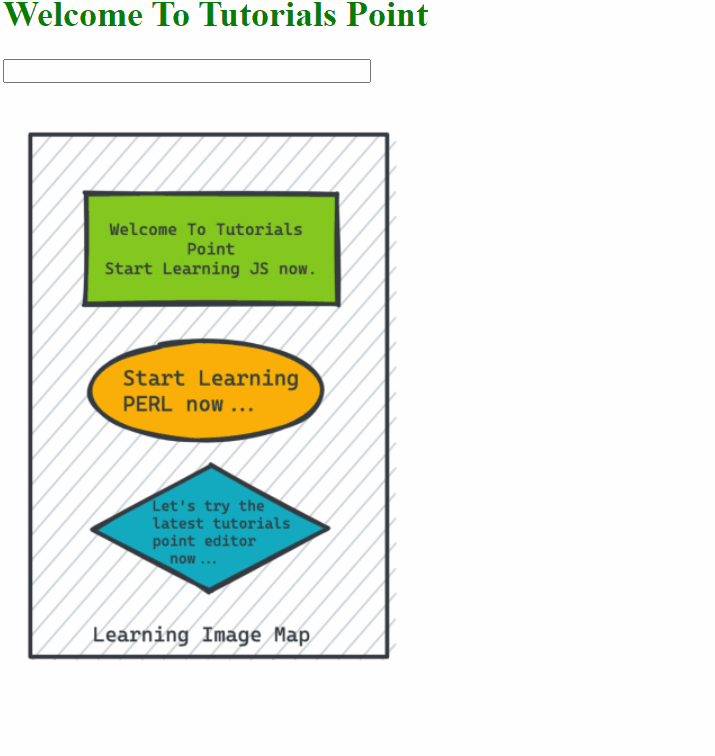# How to create an image map in JavaScript?

An Image map is a special type of image that is broken into various hotspots and each hotspot takes you to a different file. Hotspots are nothing but clickable areas upon images that are created by a user with the help of the <area> tag. This type of map is also called a client-side image map as the map is embedded in the HTML itself.

## Steps

Below is the step-by-step implementation approach on How to create an image map in JavaScript-

#### Step 1

The first step is to insert an image using the <imggt; tag. Once the image is inserted we will use an additional attribute i.e. usemap with a value that starts with #. The value of the usemap is followed by the name of the map after the #.

#### Syntax:

<img src="map.jpg" alt="sample" usemap="#mymap" width="600" height="400">

#### Step 2

Once the usemap is created we insert the image map using the <map> tag. This will create a map that is linked to the image using the required name attribute. The name attribute value must be the same as given in the usemap attribute of the <img> tag.

#### Syntax:

<map name="mymap">

#### Step 3

To create different clickable areas, we have used the tag <area>. We must define the shape of each area. The different shapes can be a rectangle, circle, and polygon. The coordinates of the area should also be defined and href is the link that will be opened when the user clicks upon the area.

#### Syntax

<area shape="rect" coords="x,y,x,y" href="">
<area shape="circle" coords="x,y,r" href="">
<area shape="poly" coords="" href="">

#### Step 4

Now for finding the coordinate of an image.

• Rectangle image will be defined with length and breadth i.e. x1, x2, y1,y2. The x1 and y1 define the coordinates on the top left corner and x2, y2 define the coordinates of the bottom right as shown in the image.

• Circle image will be defined with radius and center of the circle i.e. x, y, r where x, y defines the coordinates for the center and r defines the radius of the circle.

• Polygon image will be defined with the coordinates x1, y1, x2, y2, x3, y3, x4, y4, … where x, y defines the coordinates of one of the corners of the polygon.

## Example

In the example below, we create image map of rectangle, circle and a rhombus.

# index.html

<html>
<title>Using JavaScript Image Map</title>
<script type = "text/javascript">
<!--
function showTutorial(name) {
document.myform.stage.value = name
}
//-->
</script>
<body>
<h1 style="color: green;">elcome To Tutorials Point<h1>
<form name = "myform">
<input style="width: 50%;" type = "text" name = "stage" size = "20" />
</form>
<!-- Create Mappings -->
<img src = "https://www.tutorialspoint.com/assets/questions/media/64674/Image_map.jpg"alt = "HTML Map" border = "0" usemap = "#tutorials"/>
<map name = "tutorials">
<area shape = "rect"
coords = "74,82,298,181"
href = "https://www.tutorialspoint.com/" alt = "TutorialsPoint" target = "_self" onMouseOver = "showTutorial('Welcome to Tutorials Point')" onMouseOut = "showTutorial('')"/>
<area shape = "circle"
coords = "181,256,50"
href = "https://www.tutorialspoint.com/perl/index.htm" alt = "PERL Tutorial" target = "_self" onMouseOver = "showTutorial('PERL tutorials')" onMouseOut = "showTutorial('')"/>
<area shape="poly"
coords = "185,324,292,379,185,437,80,379"
href = "https://www.tutorialspoint.com/codingground.htm" alt = "Tutorials Point Coding Ground" target = "_self" onMouseOver = "showTutorial('Tutorials Point Coding Ground')" onMouseOut = "showTutorial('')"/>
</map>
</body>
</html>

## Output